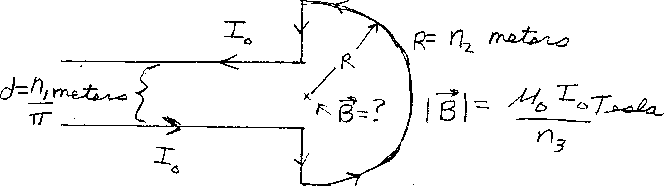Problem C14: Consider the wire shown in the figure above that has a current I0. Two sections of the wire are straight and semi-infinite in length. They are separated by a distance of n1/&pi meters. There are two other straight pieces that are connected to a semi-circular piece of radius n2 meters. What is the magnitude of the magnetic field at the center of the semi-circle? If the magnitude of the magnetic field is |B| = μ0I0/n3 Tesla, what is n3.n1 = n2 = Input n3:
If you are currently in my class, you can record your grade by entering your name and student ID number (without the leading zeros) below and clicking on "record grade".
 First Name = Last Name = ID = Problem: